Next: Solutions Up: ik Previous: Introduction

# Background

There are some substitutions in the animation process to reach simplicity in models describing the animated object. Control techniques used in inverse kinematics are often based on a skeleton consisting of links connected with joints (Figure 1). That model is usually denoted as the articulated structure'' [WW92]. There are several types of joints (revolute, prismatic, etc.) [WW92], [Mck91]. The computer animation is usually restricted to rotation joints.

The articulated models (Figure 1) have hierarchical structure where each link has its own coordinate system (CS) and is positioned relatively to the CS of the previous link. The position of the link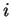in the CS of its predecessor is described by the joint angle. Thus, every joint transformation is local. That fact assists in operations with hierarchical articulated structure (Figure 1). The transformation from the CS of any given linkto the world CS is given by concatenation of partial transformations between each two neighbor links from the base to the given link.

The first link in the articulated structure is a base. The end of the structure is an end effector. The position and the orientation of the base is expressed in the global coordinates. Every articulated structure has one or more end effectors and the motion control of the structure is done through these end effectors. In many cases, the end effector takes place at the end of the structure, like palm, finger, foot, head, etc.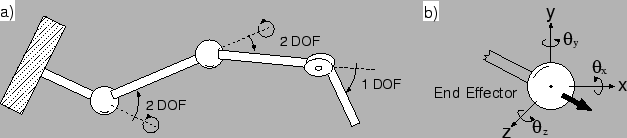The state of the articulated structure is represented by a state vector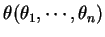in the joint (angular) space. The position and the orientation of the end effector is represented by the end effector position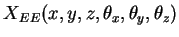in Cartesian space. The relations between the state vector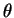and the end effector position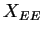are expressed by equations (1) and (2).

 Forward kinematics Inverse kinematics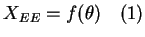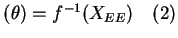The complexity of the articulated structure is expressed by the term degree of freedom (DOF). The DOF of the articulated structure is the number of independent variables necessary to specify the state of the structure.

For example, if the joint could revolute in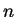axes, the degree of freedom of that joint is. When the structure (Figure 2a) consists of three joints and two of them could revolute in two axes and one could revolute in one axe, the DOF of that structure is 5.

The notation for some characteristics differs in various works. The terms in this paper are defined as follows:

State vector (angles)Transformations (generally) from link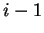to link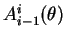Initial (base) global transformation of articulated structure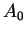Total end effector position in the global coordinates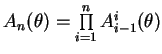As the inversion of the function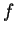is not trivial in all cases, a number of approaches solving the computation of the state vector has been proposed. The next section gives an overview of several such methods.Next: Solutions Up: ik Previous: Introduction
Lukas Barinka 2002-03-21